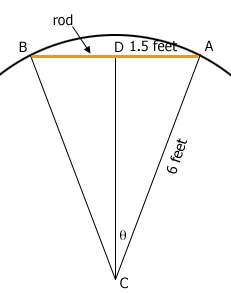SEARCH HOMEMath Central Quandaries & QueriesQuestion from Marlyn: I have a curved wall with a radius of 6'. I am trying to have a 36" rod made to hang a tapestry and need to figure out the degree measure of the arc. Can you help me please?Hi Marlyn,

I drew a diagram of what I think you are describing.$C$ is the centre of the circle, $AB$ is the rod, $D$ is the midpoint of $AB$ and $\theta$ is the measure of the angle $DCA.$$ADC$ is a right triangle so

$\tan \theta = \frac{1.5}{6}= \frac14$

and hence

$\theta = \tan^{-1} \frac14 = 14.036 \mbox{ degrees.}$

Hence the measure of the angle $BCA$ is very close to 28 degrees.

PennyMath Central is supported by the University of Regina and The Pacific Institute for the Mathematical Sciences.